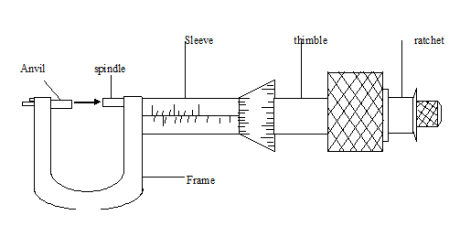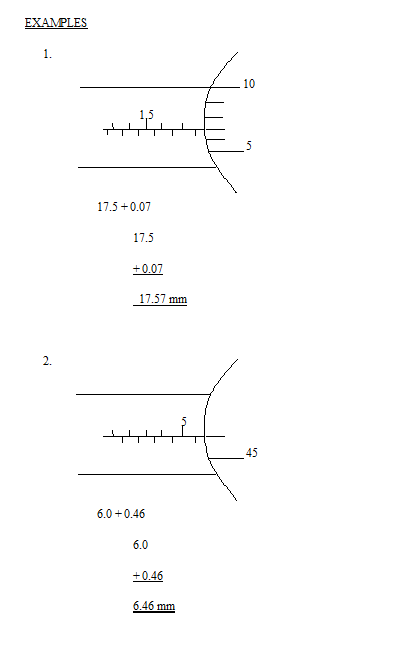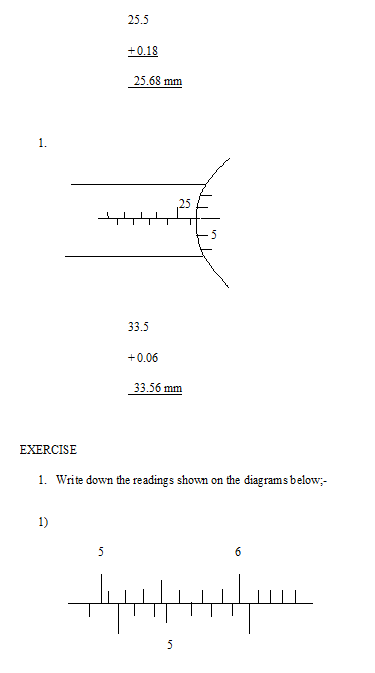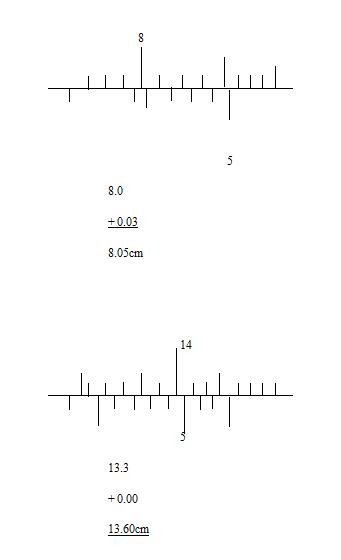PHY1: LENGTH

This Unit is about length measured in Physics olevel.

LENGTH

Length is the measurement of distance from one point to another.

The standard unit of length is a metre [m].

Other units include;-

– Kg, cm,

– Mm, dm etc

Where

1km = 1000m

1m = 1000mm

CONVERSIONS

Convert as required

1. 34m to
2. mm

1m = 1000mm

34m = 34 x 1000

= 34,000mm

1. km

1 km = 1000m

1m = 1/1000

34m = 1/1000 x 34

= 34/1000

= 0.034km

1. 04 km to mm

1km = 1,000,000mm

10.04km =  1004/100 x 1,000,000

= 10,040,000mm

1. M

1km = 1000m

10.04km = 1004/100  x 1000

= 10,040m

1. 520m to mm

1m = 1000mm

520m = 520 x 1000

= 520,000mm

1. km

1km = 1000m

= 520m

520/1000 X 520m

= 0.52km

INSTRUMENTS USED TO MEASURE LENGTH

– Tape measure

– Meter rule

– Vermeer caliper

– Micrometer screw gauge

THE METER RULE

It is used to measure straight lines, widths and heights of objects.

When using a meter rule;-

1. The rule must be in contact with the object being measured
2. The zero mark must be in line with the end of the object
• Take reading looking directly onto the mark, but not on the sidesTake readings from position B, where the eye is vertically above the mark.

When meaning length of curved objects, a string of thread will be used;

• Place one end marked with ink carefully along the curved end and mark the other and with ink. Lay the thread straight on the rule with one ink mark on the zero and reads off the second ink mark.THE MICROMETER SCREW GAUGEThis is used for measuring very small lengths such as the width of a meter rule, the diameter of a wire e.t.c

Reading is taken to the second decimal place of a millimeter [mm]. The object whose length is to be measure in fitted between the anvil and spindle by adjusting the rate let.

Precautions

Before using a micrometer screw gauge;-

I – Wipe the faces of the anvil and spindle to remove any dirt particles that may cause false readings.

II – incase of any zero error, he zero reading must be checked, corrected and correction made in the final answer.

HOW TO USE THE MICROMETER SCREW GAUGE

The object is placed between the spindle and the anvil while turning thimble.

When the spindle gets very close to the object, the ratchet is turned until a click is heard

There are two reading to take;-

Each division on the thimble represents 0.01mm on a 100 divisions micro meter screw gauge. It is accurate to 3 decimal places in cm or 2 decimal places in millimeters

VENIER CALIPERS

1. The engineer’s caliper

This is adjusted to touch the two ends of an object, and then transferred to a ruler1. The venier caliper

The venier caliper consists of two scales;-

i- Centimeter scale

ii- Millimeter scale

The scale on the form is normally in cm, while that on the sliding jaw consists of 10 divisions in mm.The venier caliper is used where a ruler may not be used e.g measuring the inner and outer diameters of round objects like a beaker, a tube , the thickness of a table e.t.c

When reading the veneir caliper, two sets of reading are considered;-

i- Reading on the main scale

ii- Reading on the venier scale

Two points on the vernier scale should be used to correct the right reading;-

i- The zero mark

ii- The point where a mark on the vernier scale coincides with the mark on the main scale

READINGS ON THE MICROMETER SCREW GAUGE

White down the readings show belowASSIGNMENT : LENGTH assignmentMARKS : 10  DURATION : 1 week, 3 days

SEE ALL
•YOU